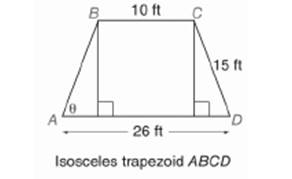Chapter 11.CR, Problem 6CR### Elementary Geometry for College St...

6th Edition
Daniel C. Alexander + 1 other
ISBN: 9781285195698

#### Solutions

Chapter
Section### Elementary Geometry for College St...

6th Edition
Daniel C. Alexander + 1 other
ISBN: 9781285195698
Textbook Problem
1 views

# In Exercises 5 to 8, state the ratio needed, and use it to find the measure of the indicated angle to the nearest degree.To determine

To find:

The measure of the angle indicated to the nearest degree.

Explanation

Procedure used:

In any right triangle ABC with mA=θ we have the following ratios.

sinθ=OppositeHypotenuse

Calculation:

Given:

Assume that the perpendicular drawn from B and C meet AD at F and E respectively.

Thus,

The above figure is a isosceles trapezium.

Hence, for

a=10 ft

b=26 ft, we have

AF=DE=12b-a

AF=DE=1226-10

AF=DE=1216

AF=DE=8 ft.

Also,

AB=CD=15 ft, and

### Still sussing out bartleby?

Check out a sample textbook solution.

See a sample solution

#### The Solution to Your Study Problems

Bartleby provides explanations to thousands of textbook problems written by our experts, many with advanced degrees!

Get Started